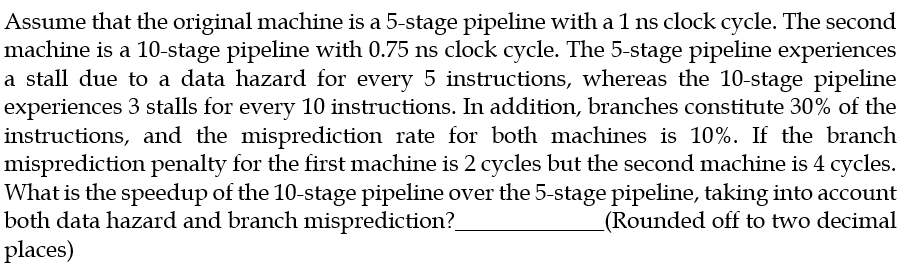115 views### 1 comment

5 stage total CPI=.2+.7+3*.03+.27*1=x1

10 stage total CPI=.3+.7+5*.03+.27=x2

devide x1 by x2 =1.18

# Check the solution here.To compare the performance of the two machines, we need to calculate the average number of cycles required to execute a single instruction on each machine.

For the 5-stage pipeline, the average number of cycles per instruction is:

1 ns + (5 instructions * 1 ns * 5% data hazard rate) + (30% branches * 1 ns * 10% misprediction rate) = 1.5 ns

For the 10-stage pipeline, the average number of cycles per instruction is:

0.75 ns + (10 instructions * 0.75 ns * 3% data hazard rate) + (30% branches * 0.75 ns * 10% misprediction rate) = 0.975 ns

The speedup of the 10-stage pipeline over the 5-stage pipeline is:

1.5 ns / 0.975 ns = 1.53

Thus, the 10-stage pipeline is approximately 1.53 times faster than the 5-stage pipeline, taking into account both data hazard and branch misprediction.

1
110 views